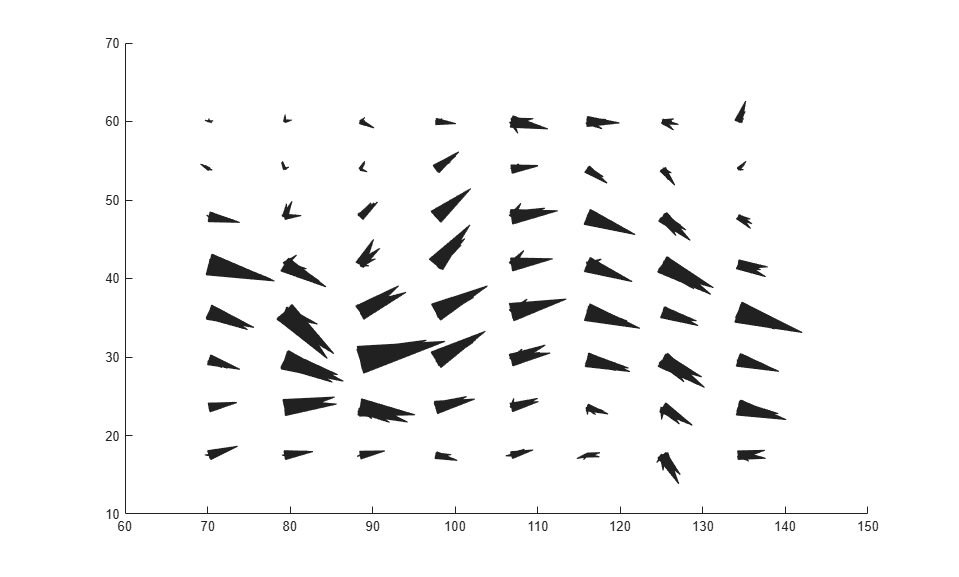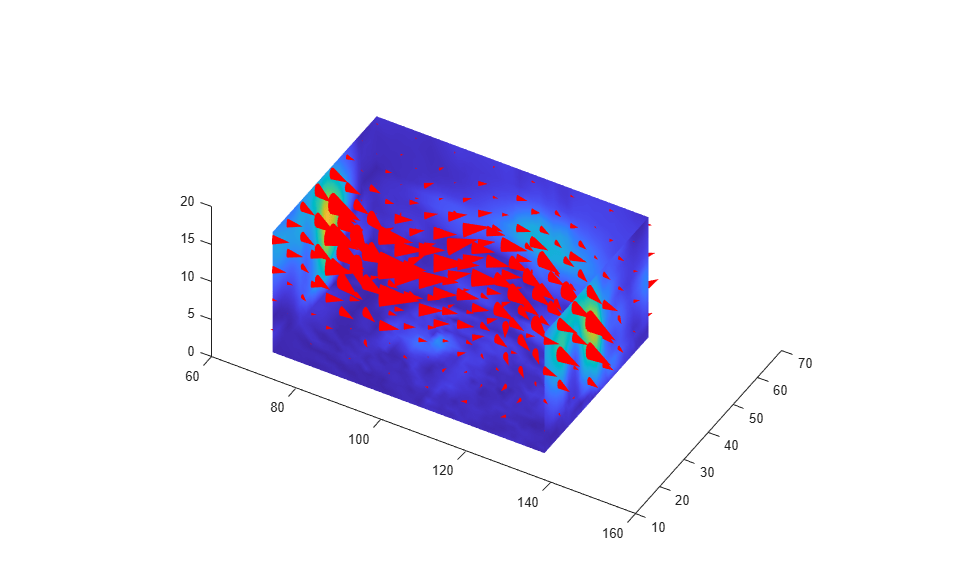# coneplot

Plot velocity vectors as cones in 3-D vector field

## Syntax

```coneplot(X,Y,Z,U,V,W,Cx,Cy,Cz) coneplot(U,V,W,Cx,Cy,Cz) coneplot(...,s) coneplot(...,color) coneplot(...,'quiver') coneplot(...,'method') coneplot(X,Y,Z,U,V,W,'nointerp') coneplot(axes_handle,...) h = coneplot(...) ```

## Description

`coneplot(X,Y,Z,U,V,W,Cx,Cy,Cz)` plots velocity vectors as cones pointing in the direction of the velocity vector and having a length proportional to the magnitude of the velocity vector. `X`, `Y`, `Z` define the coordinates for the vector field. `U`, `V`, `W` define the vector field. These arrays must be the same size, monotonic, and represent a Cartesian, axis-aligned grid (such as the data produced by `meshgrid`). `Cx`, `Cy`, `Cz` define the location of the cones in the vector field. The section Specifying Starting Points for Stream Plots in Visualization Techniques provides more information on defining starting points.

`coneplot(U,V,W,Cx,Cy,Cz)` (omitting the `X`, `Y`, and `Z` arguments) assumes `[X,Y,Z] = meshgrid(1:n,1:m,1:p)`, where ```[m,n,p]= size(U)```.

`coneplot(...,s)` automatically scales the cones to fit the graph and then stretches them by the scale factor `s`. If you do not specify a value for `s`, `coneplot` uses a value of 1. Use `s = 0` to plot the cones without automatic scaling.

`coneplot(...,color)` interpolates the array `color` onto the vector field and then colors the cones according to the interpolated values. The size of the `color` array must be the same size as the `U`, `V`, `W` arrays. This option works only with cones (that is, not with the `quiver` option).

`coneplot(...,'quiver')` draws arrows instead of cones (see `quiver3` for an illustration of a quiver plot).

`coneplot(...,'method')` specifies the interpolation method to use. `method` can be `linear`, `cubic`, or `nearest`. `linear` is the default. (See `interp3` for a discussion of these interpolation methods.)

`coneplot(X,Y,Z,U,V,W,'nointerp')` does not interpolate the positions of the cones into the volume. The cones are drawn at positions defined by `X`, `Y`, `Z` and are oriented according to `U`, `V`, `W`. Arrays `X`, `Y`, `Z`, `U`, `V`, `W` must all be the same size.

`coneplot(axes_handle,...)` plots into the axes with the handle `axes_handle` instead of into the current axes (`gca`).

`h = coneplot(...)` returns the handle to the `patch` object used to draw the cones.

`coneplot` automatically scales the cones to fit the graph, while keeping them in proportion to the respective velocity vectors.

## Examples

collapse all

Plot velocity vector cones for vector volume data representing motion of air through a rectangular region of space.

Load the data. The `wind` data set contains the arrays `u`, `v`, and `w` that specify the vector components and the arrays `x`, `y`, and `z` that specify the coordinates.

```load wind ```

Establish the range of the data to place the slice planes and to specify where you want the cone plots.

```xmin = min(x(:)); xmax = max(x(:)); ymin = min(y(:)); ymax = max(y(:)); zmin = min(z(:)); ```

Define where to plot the cones. Select the full range in `x` and `y` and select the range 3 to 15 in `z`.

```xrange = linspace(xmin,xmax,8); yrange = linspace(ymin,ymax,8); zrange = 3:4:15; [cx,cy,cz] = meshgrid(xrange,yrange,zrange); ```

Plot the cones and set the scale factor to 5 to make the cones larger than the default size.

```figure hcone = coneplot(x,y,z,u,v,w,cx,cy,cz,5); ```Set the cone colors.

```hcone.FaceColor = 'red'; hcone.EdgeColor = 'none'; ```Calculate the magnitude of the vector field (which represents wind speed) to generate scalar data for the `slice` command.

```hold on wind_speed = sqrt(u.^2 + v.^2 + w.^2); ```

Create slice planes along the x-axis at `xmin` and `xmax`, along the y-axis at `ymax`, and along the z-axis at `zmin`. Specify interpolated face color so the slice coloring indicates wind speed, and do not draw edges.

```hsurfaces = slice(x,y,z,wind_speed,[xmin,xmax],ymax,zmin); set(hsurfaces,'FaceColor','interp','EdgeColor','none') hold off ```Change the axes view and set the data aspect ratio.

```view(30,40) daspect([2,2,1]) ```Add a light source to the right of the camera and use Gouraud lighting to give the cones and slice planes a smooth, three-dimensional appearance.

```camlight right lighting gouraud set(hsurfaces,'AmbientStrength',0.6) hcone.DiffuseStrength = 0.8; ```